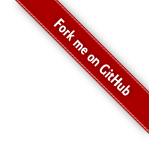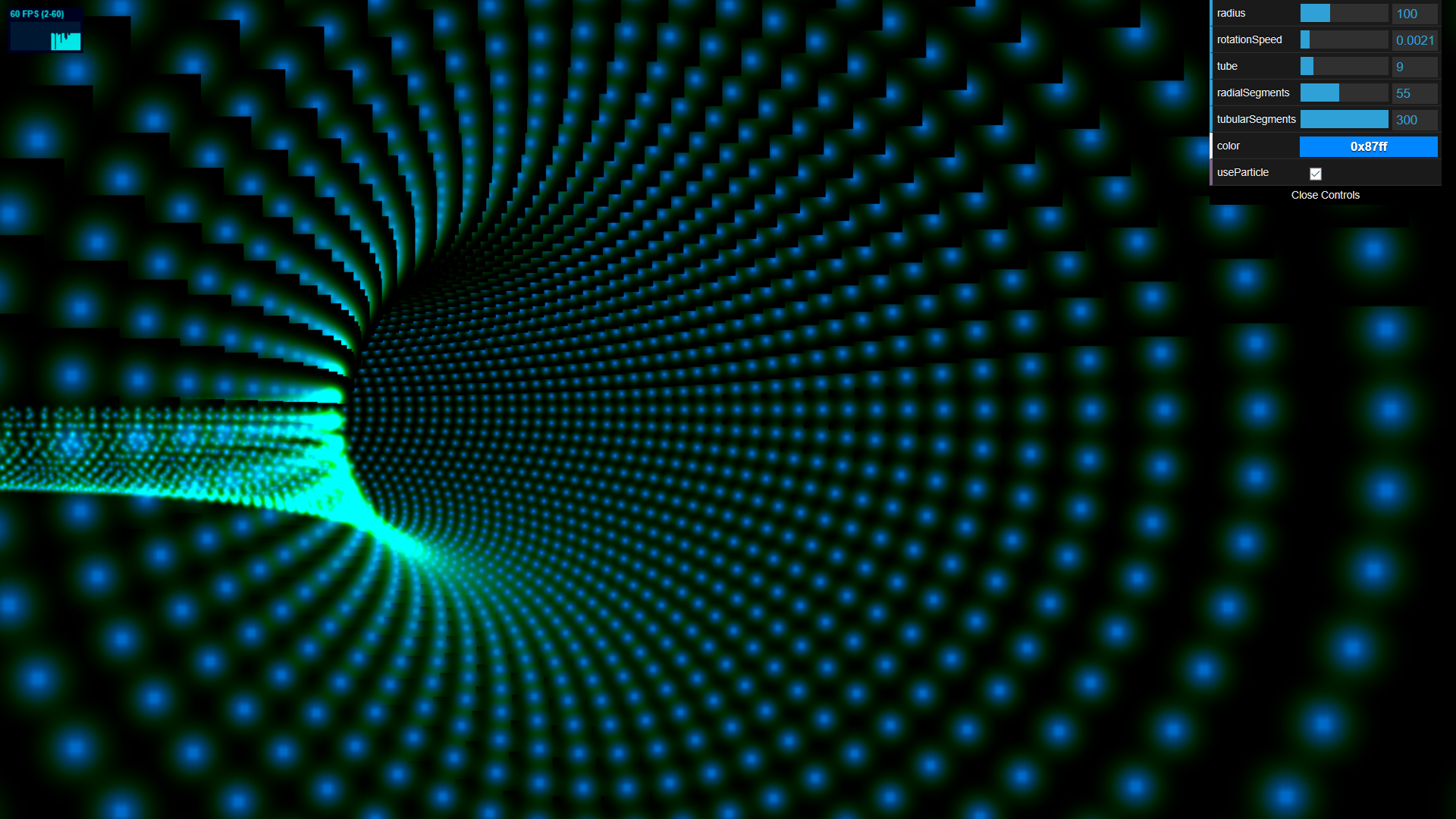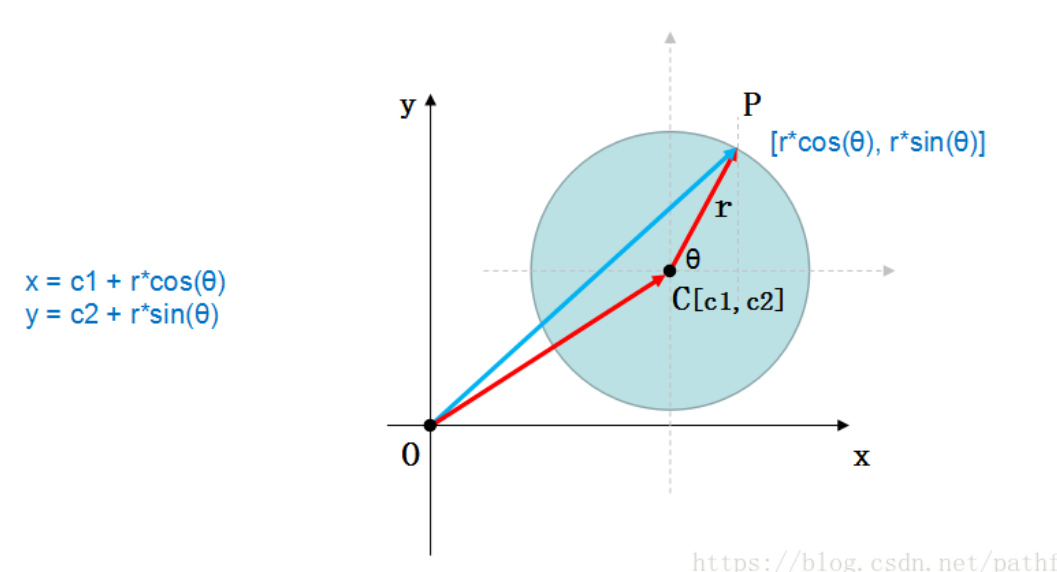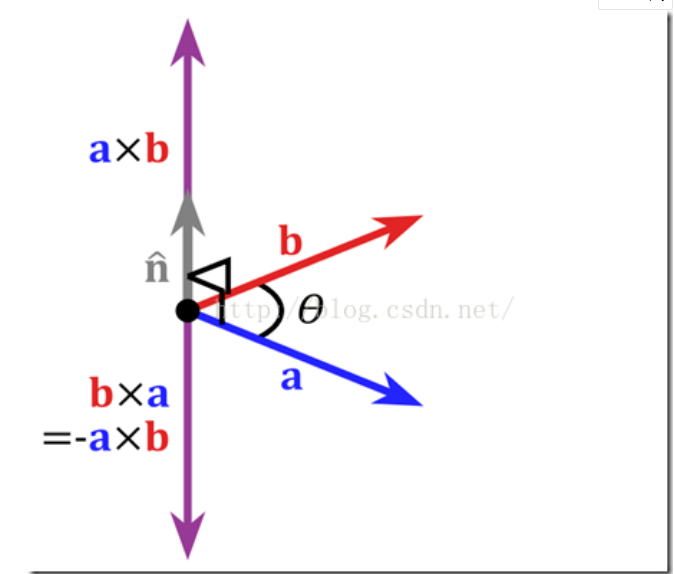WebGL three.js学习笔记 使用粒子系统模拟时空隧道（虫洞）

WebGL three.js学习笔记 使用粒子系统模拟时空隧道three.js的粒子系统

three.js的粒子系统主要是依靠精灵体来创建的，要实现three.js中的粒子系统创建，一般有两种方式。

搭建场景

let cloud = new THREE.Points(geom, material);//创建点云

,比如geometry是一个Box，那么这个点云就会有8粒子，分别分布在正方体的8个顶点上。如果我们不用geometry，我们就需要手动给点云创建很多的顶点，包括定义它们的坐标，这里我们也是用一个定义好的几何体去创建粒子。

//创建点云
function createPointCloud(geom,color) {
let material = new THREE.PointsMaterial({
color: color,
size: 3,
transparent: true,
map: generateSprite()//取得渐变的canvas纹理
});
let cloud = new THREE.Points(geom, material);//创建点云
cloud.sortParticles = true;//可以让所有粒子的Z轴得到正确摆放，不会互相遮挡
return cloud;
}

TorusGeometry的构造函数如下：
tube：管道半径
tubularSegments：管的分段数
arc：圆环面的弧度，缺省值为Math.PI * 2

let geom = new THREE.TorusGeometry(
Math.round(controls.tubularSegments)
);//TorusGeometry几何体，管道状的几何体，里面的参数设置都是菜单面板上面的参数

controls = new function () {
this.tube = 10;//管道的半径
this.tubularSegments = 200;//整个大圆隧道的段数，值越大，创造的物体更精细，也更消耗性能
this.useParticle = true;//是否使用粒子系统创造几何体
this.rotationSpeed = 0.003;//摄像机的速度
this.color = 0xffffff;//此颜色会与材质中纹理本身的颜色做乘法，最后的结果就是渲染出来的颜色
}

generateSprite函数代码（主要用到的是canvas的绘图函数，js的基础部分）：

function generateSprite() {
let canvas = document.createElement("canvas");
canvas.width = 16;
canvas.height = 16;
let context = canvas.getContext("2d");//得到canvas的绘图上下文
let gradient = context.createRadialGradient(canvas.width / 2, canvas.height / 2, 0, canvas.width / 2, canvas.height / 2, canvas.width / 2);//颜色渐变图形
context.fillRect(0, 0, canvas.width, canvas.height);

let texture = new THREE.Texture(canvas);//将得到的画好的canvas作为纹理图片
texture.needsUpdate = true;//需要设置更新，否则会没有效果
return texture;
}

this.draw = function () {
cameraInit = true;//调用此函数后，对摄像机进行一次初始化
if (obj) scene.remove(obj);//如果场景的隧道已经存在，先移除
//使用粒子系统渲染几何体
if (controls.useParticle) {
obj = createPointCloud(geom,controls.color);
obj.rotation.x = Math.PI/2;//旋转90度以后，更加方便观测
} else {//使用普通材质系统渲染几何体
obj = createMesh(geom);
obj.rotation.x = Math.PI/2;
}
}let angle = 0;//初始角度
angle = angle + controls.rotationSpeed;//相机移动的速度
//可以理解为圆形的参数方程x=rsinα，y=rcosα，function look(){
let view = new THREE.Vector3(camera.position.x,
camera.position.y,
camera.position.z);//计算当前摄像机位置点到世界中心点的向量
let vertical = (new THREE.Vector3(view.z, 0,
-1.0 * view.x)).normalize();
//两个向量的点积如果为0，则两个向量垂直，公式为x1*y2+x2*y1=0，
//这里的Y轴用Z轴代替。计算出垂直向量以后用normalize（）化成单位向量
camera.lookAt(camera.position.x+vertical.x,0,
camera.position.z+vertical.z);//camera.lookAt的值设置为 刚刚的单位向量加在当前摄像机的位置
//这样就实现了在摄像机在旋转时，一直朝前看。

}

function initThree() {
//渲染器初始化
renderer = new THREE.WebGLRenderer();
renderer.setSize(window.innerWidth, window.innerHeight);
renderer.setClearColor(0x000000);
document.getElementById("WebGL-output").appendChild(renderer.domElement);//将渲染添加到div中
//初始化摄像机，这里使用透视投影摄像机
camera = new THREE.PerspectiveCamera(50, window.innerWidth / window.innerHeight, 0.1, 1000);
camera.up.x = 0;//设置摄像机的上方向为哪个方向，这里定义摄像的上方为Y轴正方向
camera.up.y = 1;
camera.up.z = 0;
look();//计算摄像机在当前位置应该对准的目标点，即camera.lookAt的设置

//初始化场景
scene = new THREE.Scene();

}

完整的代码如下：

<!DOCTYPE html>
<html lang="en">
<meta charset="UTF-8">
<title>Sprite Tunnel</title>
<script src="../../import/three.js"></script>
<script src="../../import/stats.js"></script>
<script src="../../import/Setting.js"></script>
<script src="../../import/dat.gui.min.js"></script>
<style type="text/css">
div#WebGL-output {
border: none;
cursor: pointer;
width: 100%;
height: 850px;
background-color: #000000;
}
</style>
<div id="WebGL-output"></div>
<script>
let camera, renderer, scene;
let controls;

function initThree() {
//渲染器初始化
renderer = new THREE.WebGLRenderer();
renderer.setSize(window.innerWidth, window.innerHeight);
renderer.setClearColor(0x000000);
document.getElementById("WebGL-output").appendChild(renderer.domElement);//将渲染添加到div中
//初始化摄像机，这里使用透视投影摄像机
camera = new THREE.PerspectiveCamera(50, window.innerWidth / window.innerHeight, 0.1, 1000);
camera.up.x = 0;//设置摄像机的上方向为哪个方向，这里定义摄像的上方为Y轴正方向
camera.up.y = 1;
camera.up.z = 0;
look();//计算摄像机在当前位置应该对准的目标点，即camera.lookAt的设置

//初始化场景
scene = new THREE.Scene();

}
//计算摄像机在当前位置应该对准的目标点
function look(){
let view = new THREE.Vector3(camera.position.x, camera.position.y, camera.position.z);//计算当前摄像机位置点到世界中心点的向量
let vertical = (new THREE.Vector3(view.z, 0, -1.0 * view.x)).normalize();//两个向量的点积如果为0，则两个向量垂直，公式为x1*y2+x2*y1=0，这里的Y轴用Z轴代替。计算出垂直向量以后用normalize（）化成单位向量
camera.lookAt(camera.position.x+vertical.x,0,camera.position.z+vertical.z);//camera.lookAt的值设置为 刚刚的单位向量加在当前摄像机的位置，这样就实现了在摄像机在旋转时，一直朝前看。

}
//
let obj;
let cameraInit = false;//改动隧道的半径后，需要让摄像机重新初始化，当cameraInit为true时进行初始化，先定义为false
//初始化菜单面板
function initDatGUI() {
//设置菜单中需要的参数
controls = new function () {
this.tube = 10;//管道的半径
this.tubularSegments = 200;//整个大圆隧道的段数，值越大，创造的物体更精细，也更消耗性能
this.useParticle = true;//是否使用粒子系统创造几何体
this.rotationSpeed = 0.003;//摄像机的速度
this.color = 0xffffff;//此颜色会与材质中纹理本身的颜色做乘法，最后的结果就是渲染出来的颜色
//初始化渲染场景中的隧道以及粒子系统的函数
this.draw = function () {
cameraInit = true;//调用此函数后，对摄像机进行一次初始化
if (obj) scene.remove(obj);//如果场景的隧道已经存在，先移除
//使用粒子系统渲染几何体
if (controls.useParticle) {
obj = createPointCloud(geom,controls.color);
obj.rotation.x = Math.PI/2;//旋转90度以后，更加方便观测
} else {//使用普通材质系统渲染几何体
obj = createMesh(geom);
obj.rotation.x = Math.PI/2;
}
}
};
let gui = new dat.GUI();
//将刚刚设置的参数添加到菜单中

//这里需要先调用一次draw()函数，否则刚开始的时候会没有东西背渲染出来
controls.draw();
}
//精灵贴图的制作，场景的粒子系统的每一个粒子都用这里制作的贴图来模拟
function generateSprite() {
let canvas = document.createElement("canvas");
canvas.width = 16;
canvas.height = 16;
let context = canvas.getContext("2d");//得到canvas的绘图上下文
let gradient = context.createRadialGradient(canvas.width / 2, canvas.height / 2, 0, canvas.width / 2, canvas.height / 2, canvas.width / 2);//颜色渐变图形
context.fillRect(0, 0, canvas.width, canvas.height);

let texture = new THREE.Texture(canvas);//将得到的画好的canvas作为纹理图片
texture.needsUpdate = true;//需要设置更新，否则会没有效果
return texture;
}
//创建点云
function createPointCloud(geom,color) {
let material = new THREE.PointsMaterial({
color: color,
size: 3,
transparent: true,
map: generateSprite()//取得渐变的canvas纹理
});
let cloud = new THREE.Points(geom, material);//创建点云
cloud.sortParticles = true;//可以让所有粒子的Z轴得到正确摆放，不会互相遮挡
return cloud;
}
//创建普通的管道几何体
function createMesh(geom) {
let material = new THREE.MeshNormalMaterial();
material.side = THREE.DoubleSide;//双边渲染
let mesh = new THREE.Mesh(geom, material);
return mesh;
}

let angle = 0;//初始角度
//渲染函数
function render() {
if(cameraInit){//每次重新渲染场景的时候，重新设置相机的位置与角度
angle = 0;
cameraInit=false;
}
angle = angle + controls.rotationSpeed;//相机移动的速度
look();
stats.update();
renderer.clear();
requestAnimationFrame(render);
renderer.render(scene, camera);
}

//功能函数
function setting() {
</html>